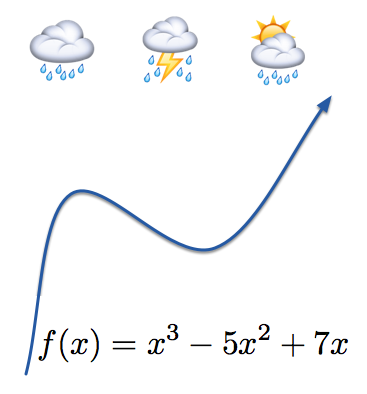# Raining in Flatland

Calculus Level 3In flatland, everything is two-dimensional. There is a container with the shape of a curve

$f(x)={ x }^{ 3 }-5{ x }^{ 2 }+7x.$

If gravitational force is parallel to $y$-axis, what is the maximum amount of water it can contain?

Give your answer to 3 decimal places.

×

Problem Loading...

Note Loading...

Set Loading...